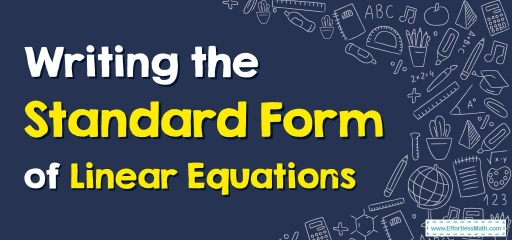# How to Write the Standard Form of Linear Equations?

The standard form of linear equations is a way of writing linear equations. In this guide, you learn more about writing the standard form of linear equations.A linear equation can be written in various forms such as the standard form, the slope-intercept form, and the point-slope form. The standard form of linear equations is also known as the general form.

## A step-by-step guide to the standard form of linear equations

The equation in which the highest power of the variable is $$1$$ is called the linear equation or the one-degree equation. The standard form of the linear equation is shown as follows:

$$\color{blue}{Ax + By = C}$$

where $$A, B$$, and $$C$$ are integers, and the letters $$x$$ and $$y$$ are the variables.

### The standard form of linear equations in one variable

A linear equation in one variable means that the equation contains only one variable. That is, this linear equation has one solution to it. The standard form or general form of linear equations in one variable is written as follows:

$$\color{blue}{Ax + B = 0}$$

Where:

• $$A$$ and $$B$$ are integers
• $$x$$ is the single variable

### The standard form of linear equations in two variables

When a linear equation has two variables, it has two solutions. The standard form of linear equations (a general form of linear equations) in two variables is expressed as follows:

$$\color{blue}{Ax+By=C}$$

Where:

• $$A, B$$, and $$C$$ are integers
• $$x$$ and $$y$$ are the variables

### How to write the standard form of linear equations in two variables?

When we need to rewrite a linear equation to its standard form, we can easily convert it to a general form, $$Ax + By = C$$, where $$A, B,$$ and $$C$$ must be integers, and the order of the terms should be as given.

### Standard Form of Linear Equations– Example 1:

Rewrite the linear equation in standard form, $$3y=-6x+9$$.

Solution:

To rewrite the given equation in the standard form, move the expression $$-6x$$ to the left. This means it will become $$3y+6x=9$$. we can arrange the terms on the left-hand side as per the order given in the standard form. This will make it $$6x+3y=9$$.

## Exercises forStandard Form of Linear Equations

### Rewrite the linear equation in standard form.

1. $$\color{blue}{-y=4x+8}$$
2. $$\color{blue}{y=7x}$$
3. $$\color{blue}{3x^2+9=15}$$
4. $$\color{blue}{-\frac{2}{3}x-\frac{5}{2}y=4}$$
1. $$\color{blue}{4x+y=-8}$$
2. $$\color{blue}{7x-y=0}$$
3. $$\color{blue}{Not\:a\:linear\:equation}$$
4. $$\color{blue}{4x+15y=-24}$$

### What people say about "How to Write the Standard Form of Linear Equations? - Effortless Math: We Help Students Learn to LOVE Mathematics"?

No one replied yet.

X
30% OFF

Limited time only!

Save Over 30%

SAVE $5 It was$16.99 now it is \$11.99